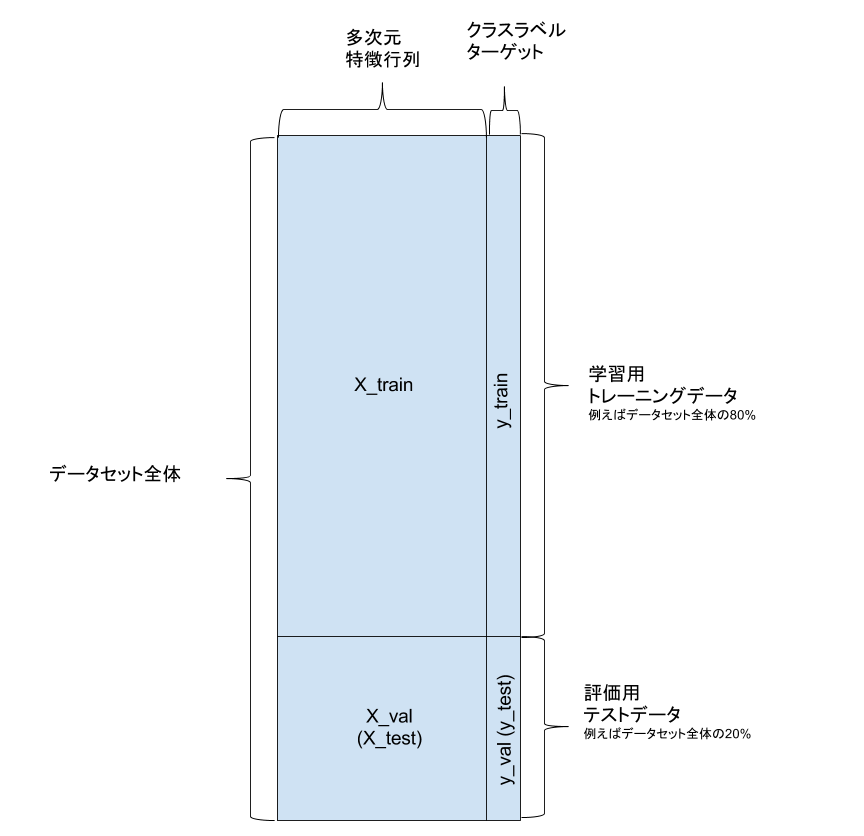# [PYTHON] Machine learning Training data division and learning / prediction / verification

When studying or teaching machine learning based on PyData.Tokyo Tutorial # 1, from the division of training data, I find it difficult to understand the learning, prediction, and verification parts. I will explain this part.

# Prerequisites

--Supervised learning-> In other words, there is labeled data --There are a certain number of datasets-> 890 in this tutorial --Learning and verifying with 20% of test data left --The feature matrix is multidimensional (it is natural ...) --Use sklearn (scikit-learn) --Estimate by logistic regression --See `pydatatokyo_tutorial_ml.ipynb` in PyData.Tokyo Tutorial # 1 for detailed code.

# Training data split

Feature matrix `X` Class label data `y` If you do the following, you can divide the data.

``````from sklearn.model_selection import train_test_split
X_train, X_val, y_train, y_val = train_test_split(X, y, train_size=0.8, random_state=1)
``````--X_train: Feature matrix for learning (80%) --X_val: Evaluation feature matrix (20%) --y_train: Training class label (80%) Unknown data --y_val: Evaluation class label (20%) Used for answering unknown data (keep it hidden)

# Learning / prediction / verification

## Initialization of classifier (learner)

``````from sklearn.linear_model import LogisticRegression
clf = LogisticRegression()
``````

Initialize clf and use it for the following learning, prediction, and verification.

## Learning

``````clf.fit(X_train, y_train)
``````

Train using the initialized clf fit method The data gives 80% of the training data a feature matrix and class labels

## Forecast

``````y_train_pred = clf.predict(X_train)
y_val_pred = clf.predict(X_val)
``````

Predict with clf's predict method.

--`y_train_pred`: Result of re-prediction with training data --`y_val_pred`: Result of prediction using evaluation data

So far, I haven't used `y_val`. That is, `y_train` is treated as unknown data

## Evaluation / verification

``````from sklearn.metrics import accuracy_score
train_score = accuracy_score(y_train, y_train_pred)
val_score = accuracy_score(y_val, y_val_pred)
``````

ʻAccuracy_score` is given` class label data`and`predicted result` above, and the correct answer rate is output.

--train_score: Results of prediction using training data --val_score: As a result of making a prediction using evaluation data, it means that a prediction was made using unknown data.